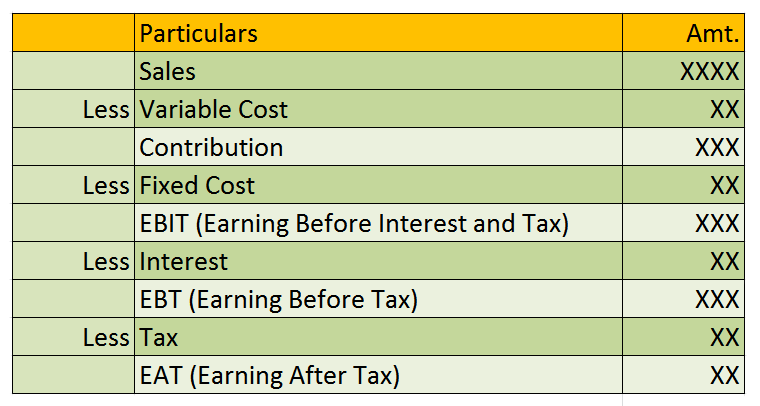# Operating and Financial Leverage Formula

Posted on

by

Leverage is financial term which used to describes the firm’s ability to use the fixed cost assets or fund to magnify the returns to its owners. There are two major and one lest known and used leverages which are degree of operating leverage and degree of financial leverage or combined leverage. Let’s see all of them in detail.## Operating Leverage

Operating leverage is concerned with the operation of the firm. Operating leverage relates to the sales and profit variations. Operating leverage is determined by the relationship between sales revenue and earning before interest and tax. Operating leverage is the firm’s ability to use the fixed operating costs to magnify the effects of changes in sales on its earning before interest and takes. The following is the formula to calculate the degree of operating leverage.

Degree of Operating Leverage = Contribution / EBIT

## Financial Leverage

Financial leverage is concerned with the effect on earning due to rise of fixed cost funds. Financial leverage arises only when the firm employs debt funds with fixed charge. Financial leverage represents the relationship between the firms earning before interest and taxes and the effect of change in earnings before interest and taxon the earning per share. There will be no financial leverage if there is no fixed charged financing. The following is the formula to calculate the degree of financial leverage.

Degree of Financial Leverage = EBIT / EBT

## Combined Leverage

Combine leverage is combination of operating as well as financial leverage. The degree of combined leverage measures the rate of change in EPS (Earning Per Share) due to change in the Sales. The following is the formula to calculate the degree of combined leverage.

Degree of Combined Leverage = Operating Leverage * Financial Leverage

or

Degree of Combined Leverage = Contribution / EBT

These are all about leverages formula used in financial management.

Read related contents by similar tags: